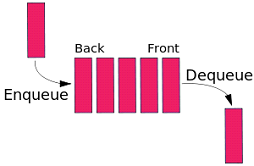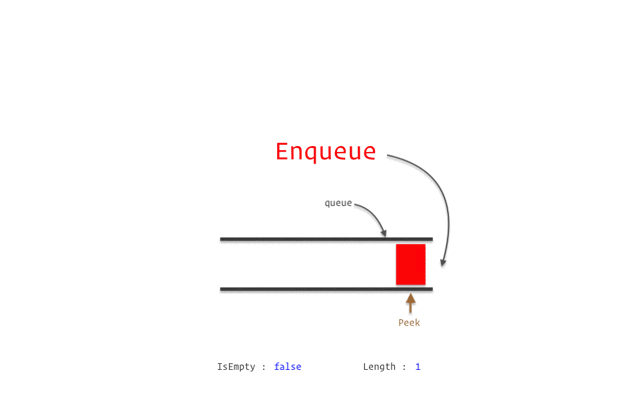## DEV Community

Damian

Posted on • Originally published at overthinkbox.com on

# Queues

A queue is a simple data structure that allows elements to be inserted from one end, called the rear (also called tail), and deleted from the other end, called the front (also called head).A queue is a collection of items that obeys the principle of first-in/first-out. It is a method for handling data structures where the first element is processed first and the newest element is processed last.

## How Implement a Queue ?

Implementing a queue will probably lead us to have some methods linked such as get the size, adding a new element, deleting an element, or simply knowing if it is empty. Always respecting the order of implementation on which this type of data structure mentioned above (FIFO) is based.### Let's do some code

First we need a function to create our queue, right ? So let's create a new function using the native methods of JS (We are doing something simple to get the concept)

``````function createQueue() {
const queue = [];
return queue;
}
``````

So far we have a function that returns an empty array. But we want to add some functionality to the implementation. For example, let's queue an item in our new queue

``````function enqueue() {
queue.unshift(item);
}
``````

What's going on here ? When we call our enqueue method, the unshift method, add whatever element we indicate to it, at the beginning of the queue.

Reference to Unshift Method

Now we can queue methods, but let's go further and add a method to unqueue

``````function dequeue() {
return queue.pop();
}
``````

As we said before, this type of structure is commonly called FIFO, so we need to remove the last item that we enter, that is what the native pop function of arrays in JS takes care of.

Reference to Pop Method

Our structure is almost ready, now let's add two methods to calculate the current size of our queue and check if it is empty.

``````function length() {
return queue.length
}
function isEmpty() {
return queue.length == 0
}```

We will use the native method length, to calculate the dimension of our queue. And we will do a simple mathematical check equaling 0 to know if our queue is empty or not.

Now, let's put it all together.

```javascript
function createQueue() {
const queue = [];
return {
enqueue(item) {
queue.unshift(item);
},
dequeue() {
return queue.pop();
},
get length() {
return queue.length;
},
get values() {
return queue;
},
isEmpty() {
return queue.length == 0;
}
};
}

const myQueue = createQueue(); console.log("Is Empty ?", myQueue.isEmpty()); // true
myQueue.enqueue("Adding my first element"); myQueue.enqueue("Learning how a queue works");

console.log("My Queue Elements", myQueue.values); // ["Learning how a queue works", "Adding my first element"]
console.log("Is Empty ?", myQueue.isEmpty()); // false console.log("Size of Queue", myQueue.length); // 2

myQueue.dequeue();
console.log("Size of Queue", myQueue.length); // 1

``````

### Real Life Uses

• Queue is used in BFS (Breadth First Search) algorithm. It helps in traversing a tree or graph.
• Queue is also used by Operating systems for job scheduling.
• Queue is used in networking to handle congestion.

Really Funny Example of a Queue

What I need to create my own Snake “Nokia” game ?

Okay, so initially my snake will be of some X pixels length. Every time it consumes food, its length increments by 1. I’ll also have to keep track of the snake’s head, or the start. And the end. And also any turning points on the body. And every time the user turns the snake in a valid direction, I’ll have to add a new turning point at that position. And then the tail — the end of the snake has to follow the same turns the head made initially. So let me rephrase that. The head first turns. Eventually the rest of the body turns. The head turns again, the body turns again. If head turns East then North then West, the body turns East then North then West. I’ll need a queue.

## Bonus

Priority Queue

Priority Queue is an extension of queue with following properties:

• Every item has a priority associated with it.
• An element with high priority is dequeued before an element with low priority.
• If two elements have the same priority, they are served according to their order in the queue.
• In the below priority queue, element with maximum ASCII value will have the highest priority.

Enqueue

Our method for enqueue items will now receive a second argument, which will tell us if the item has a high priority. By default this value will be false. Because we could omit the value if we do not want to indicate if it has a high priority. In this way, according to the conditional logic that we apply, the item will be added to the low priority queue.

``````function enqueue(item, isHighPriority = false) {
isHighPriority
? highPriorityQueue.enqueue(item)
: lowPriorityQueue.enqueue(item);
}```

*Dequeue*

Our method for dequeue will be set first in the high priority list, in case it is not empty, dequeue the first item in the high priority list. Otherwise go to the low priority list to remove an item.

```javascript
function dequeue() {
if (!highPriorityQueue.isEmpty()) {
return highPriorityQueue.dequeue();\
}

return lowPriorityQueue.dequeue(); }
``````

Peek

Our peek method gets a similar change. Just like we dequeue from the high priority queue first, we're going to peek from the high priority queue first as well. In fact, I can copy-paste this code and just make a change to which method is being called.

``````function peek() {
if (!highPriorityQueue.isEmpty()) {
return highPriorityQueue.peek();
}

return lowPriorityQueue.peek(); }
``````

Length

Our length method will only return the size of both queues added together.

``````function length() {
return highPriorityQueue.length + lowPriorityQueue.length;
}```

*Is Empty*

Lastly, our isEmpty method is the conjunction of the two queues' isEmpty methods.

```javascript
function isEmpty() {
return highPriorityQueue.isEmpty()
&& lowPriorityQueue.isEmpty();
}```

Let's put all together

```javascript
import { createQueue } from "./queue";

function createPriorityQueue() {
const lowPriorityQueue = createQueue();
const highPriorityQueue = createQueue();

return {
enqueue(item, isHighPriority = false) {
isHighPriority
? highPriorityQueue.enqueue(item)
: lowPriorityQueue.enqueue(item);
},
dequeue() {
if (!highPriorityQueue.isEmpty()) {
return highPriorityQueue.dequeue();
}

return lowPriorityQueue.dequeue();
},
peek() {
if (!highPriorityQueue.isEmpty()) {
return highPriorityQueue.peek();
}

return lowPriorityQueue.peek();
},
length() {
return highPriorityQueue.length + lowPriorityQueue.length;\
},
isEmpty() {
return highPriorityQueue.isEmpty()
&& lowPriorityQueue.isEmpty();
}
};
}

const myQueue = createPriorityQueue();

myQueue.enqueue("A fix here");
myQueue.enqueue("A bug there");
myQueue.enqueue("A new feature");

console.log(myQueue.peek()); // A fix here

myQueue.dequeue();

console.log(myQueue.peek()); // A bug there

console.log(myQueue.peek()); // Emergency task! myQueue.dequeue(); console.log(myQueue.peek()); // A bug there
``````

Play Code

### Real Life Uses

• Dijkstra’s Shortest Path Algorithm - Prim’s algorithm - Huffman codes for Data compression.
• Heap sort